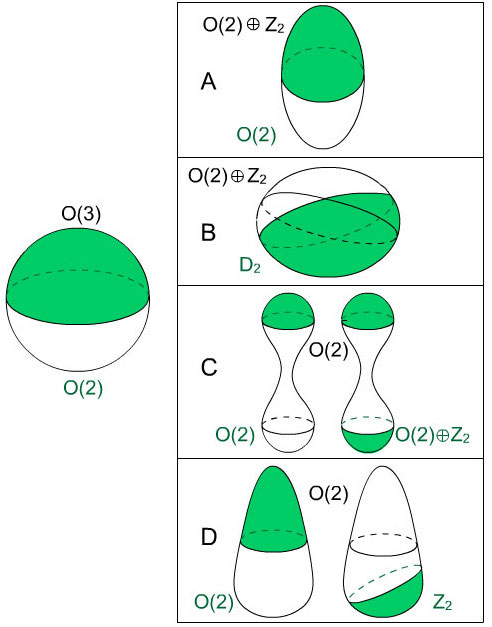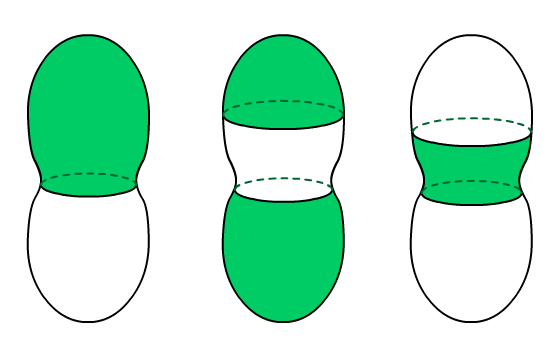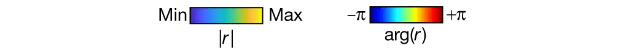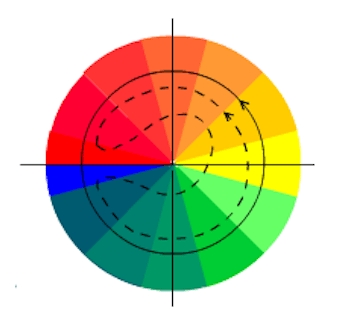# Tony’s Take October 2022

### “Against Algebra” in The Atlantic.

Temple Grandin, a professor of animal science at Colorado State, is famous for being a high-functioning autist who used her extra sensitivity to intuit how animals react to stimuli and made our handling of livestock more humane and more efficient. In her “Against Algebra” article in The Atlantic (October 6, 2022) she laments the way elementary and high-school education has evolved towards material that can be tested with numerical scores (preferably the product of machine grading) and away from more hands-on activities — she mentions how when she went to school, shop class was “the highlight of [her] day.”

Grandin reminds us that people don’t all think the same way. Some are “visual thinkers” and some are more language-oriented. And among the visual thinkers she distinguishes the “object visualizers,” who process raw visual data (these people end up as designers or mechanical engineers) from the “spatial visualizers” whose perception filters through patterns and abstractions (here we have musicians, computer people and mathematicians). The problem she sees in education today, and particularly in math education, is that it privileges the last group and has little to offer the others.

The main culprit is Algebra. A typical curriculum for Algebra I is almost completely abstract. According to Grandin, this is both unnecessary and inappropriate. Unnecessary because many of the courses or career paths that require competence in Algebra as a prerequisite don’t actually need it. As she puts it: “I teach veterinarians, but I couldn’t get into veterinary school myself, because I couldn’t do the math.” Inappropriate because the cognitive skills necessary for handling abstract reasoning often mature later in adolescence.

Grandin remarks that in 2017 (well before the COVID-19 pandemic) two thirds of students entering community college and one third of those entering four-year schools required remedial math. She suggests that “maybe the decline in performance points to a deficiency not so much in how well students master material, but in what we are asking them to master.”

I think a better approach would have been “Against how Algebra is taught in schools today.” Grandin teaches veterinarians, but she is not responsible for calculating, for example, correct dosage based on an animal’s weight and age. Students need to learn how to understand and manipulate mathematical expressions. On the other hand, the “one size fits all” curriculum and teaching methodology that she decries is clearly not working for many of them. The waste of human potential is indeed scandalous, but remedies will be difficult and expensive.

—TP

### Symmetry groups, curvature and biological cell polarization.

“Forced and spontaneous symmetry breaking in cell polarization” ran in the August 2022 issue of Nature Computational Science. As authors Pearson Miller, Daniel Fortunato and Stanislav Shvatsman of the Flatiron Institute, NYC and their collaborators explain, cell polarity — the presence of a directional axis in each cell — is essential, for example, in establishing the direction in which a cell will move or grow. In this article the authors explain how in some cases, a cell’s polarization can be determined by its shape. For computational efficiency, they restrict themselves to shapes that are axisymmetric, i.e., are invariant under rotation about an axis.

They model the process with a solid shape enclosed by a boundary, where a single chemical species is located on the boundary or in the bulk. They posit that this chemical undergoes chemical reactions and also diffuses throughout the shape in a reaction-diffusion process (like those identified by Turing and that lead to stripes and spots on animal’s coats). Among other requirements, the diffusion is substantially faster in the interior than on the boundary. This leads to a set of equations that they solve numerically to determine steady-state solutions for various cell shapes. The results of the numerical experiments are summarized in this image, adapted from the article.Chemical concentration is schematically represented in green. Each of the axisymmetric shapes derived from the most symmetrical one (the sphere, center) is labeled in black with its own symmetry group (details below) and in green with the symmetry group of the final distribution of the chemical. In most cases, the green group is strictly smaller: the initial symmetry has been broken. Adapted from Miller, P.W., Fortunato, D., Muratov, C. et al. Forced and spontaneous symmetry breaking in cell polarization. Nat Comput Sci 2, 504–511 (2022). https://doi.org/10.1038/s43588-022-00295-0

Symmetry groups. Symmetry groups allow us to quantify exactly how symmetrical an object or a configuration is. The symmetry group of an object is the set of all rigid motions (rotations and/or reflections) that leave it looking the same. It is a group because the composition of two rigid motions (performing one after the other) is again a rigid motion. This illustration uses standard mathematical symbols for the symmetry groups that appear. Shown in the center, a round sphere is symmetric under any origin-preserving rotation or reflection of 3-space. The group of these rigid motions is the 3-dimensional orthogonal group O$(3)$. The final distribution of the chemical has polarized the sphere so that only rigid motions that preserve the vertical axis leave the configuration unchanged. The broken symmetry group is the 2-dimensional orthogonal group O$(2)$.

If instead the cell has the vertically stretched (“prolate”) shape A, then its symmetries are the axis-preserving O$(2)$ plus up-down flips. Since flipping twice gets you back to where you started, the flips can be represented by the group $Z_2$ of integers mod 2, where $1+1=0$; since the flips and O$(2)$ operate independently, the complete symmetry group of the prolate sphere is the direct sum O$(2)\oplus Z_2$. Here the final distribution of the chemical occurs at one of the geometrical poles, so the flip symmetry is broken.

If the sphere is vertically squashed (“oblate”) the new shape (B) again has symmetry group O$(2)\oplus Z_2$, but the final chemical distribution will be centered about a point on the equator. This configuration is not preserved by rotations, but by up-down flips and front-back flips. These form the symmetry group $D_2$ of a non-square rectangle.

Pinching the sphere in its center (shape C) yields another solid with symmetry group O$(2)\oplus Z_2$; in this case there are two possible final distributions of the chemical, one of which admits the full symmetry group of the solid. Also, if the sphere is deformed to the cone-like shape D, there are two possible outcomes; one has the axis-preserving symmetry group O$(2)$, but the other only allows back-to-front flips, hence $Z_2$.

In the patterns shown in the figure above, the high-concentration area centers about a point (or points) where the Gaussian curvature has a local maximum, in the sense that it is greater than or equal to the curvature at neighboring points.

But if the surface also has areas of negative curvature, it is a different story.The surface of the axially symmetric peanut has regions of both positive and negative Gaussian curvature. Such surfaces, the authors tell us, “can admit a number of solutions, including multiple stable caps and solutions where high concentrationdoes not localize to high positive curvature.” Image adapted from Miller, P.W., Fortunato, D., Muratov, C. et al. Forced and spontaneous symmetry breaking in cell polarization. Nat Comput Sci 2, 504–511 (2022). https://doi.org/10.1038/s43588-022-00295-0.

### Mathematical singularities and optical vortices.

“Spontaneous generation and active manipulation of real-space optical vortices” by Dongha Kim, Min-kyo Seo and collaborators at the Korea Advanced Institute of Science and Technology ran in Nature on October 12, 2022. Optical vortices are “beams of light that carry angular momentum.” Kim and collaborators demonstrate a way of producing oppositely-oriented pairs of vortices using reflection from a multilayered sandwich of materials called a gradient thickness optical cavity (GTOC). The vortices occur in the space just above the sandwich; they are due to interference between light rays reflected off the top of the nickel layer (shown dark blue in the diagram below) and rays which traverse that layer and are reflected off the aluminum mirror at the bottom. That interference pattern varies from point to point across the surface because the thickness of the upper silicon dioxide layer (shown light blue) increases from front to back, while that of the lower SiO$_2$ layer increases from right to left. Vortices only occur when the optical thickness of the nickel layer (which is controlled by an exterior magnetic field) is in a certain range, and then they appear and disappear as that thickness is varied.A schematic illustration of the experimental set-up. The sandwich has a reflective aluminum layer on the bottom. Above it are two silicon dioxide layers separated by a nickel layer whose optical thickness $h_{Ni}$ can be controlled by an external magnetic field. The SiO$_2$ layers have thickness $h_1$ and $h_2$ varying linearly across the surface, in such a way that the gradients $\nabla h_1$ and $\nabla h_2$ are orthogonal. The inset graphs show the magnitude $|r|$ and phase $\arg(r)$ of the complex reflection coefficient as a function of $h_1$ and $h_2$ (and therefore, locally, of position on the surface of the GTOC). Image courtesy of Dongha Kim.The magneto-optic effect. As the optical height of the nickel layer is increased, the initial reflectance minimum splits into two minima with oppositely oriented local topology. Image courtesy of Dongha Kim.

Some details: the sandwich is about 2cm square; the gradients of $h_1$ and $h_2$ are on the order of one in a million. The nickel layer is only 5 nm thick, but varying an external magnetic field can give it optical thickness up to 20nm. The phenomenon described in the article occurs only when that thickness is in the range approximately 6 to 13 nm. In general, the reflective point-by-point response of the sandwich to the external magnetic field repeats 2-dimensionally (the period depends on the wavelength of the incident light and on the refractive index of SiO$_2$).

As the authors explain in the abstract, “the vortex–antivortex pairs present in the light reflected by our device are generated through mathematical singularities in the generalized parameter space of the top and bottom silicon dioxide layers.” The structure of the combined reflected rays is in fact characterized by the complex reflection coefficient function. Mathematically speaking, this is a complex-valued function defined for points on the surface of the sandwich (and therefore for pairs $h_1, h_2$ of layer depths), and vortices correspond to singularities of this function. The behavior of the complex reflection coefficient function near a positive minimum in its absolute value (“trivial” case) and near a pair of singularities (“non-trivial” case) is represented in the inset graphs in the illustrations of the experiment, above. A complex function would require a 4-dimensional graph; writing a complex number in polar notation as $re^{i\theta}$ the authors have represented the function by two ordinary graphs, one for the absolute value $r$, and one for the argument $\theta$. Imagine tracking the phase of the combined reflected beam around a loop enclosing the minimum; this is its argument when its intensity is written as a complex number. The lengths of the optical paths traversed by the two reflected beams vary from point to point, and consequently so does the phase of their sum. For special values of the optical thickness of the nickel layer, at the end of the loop the accumulated phase change is exactly $2\pi$. This spiraling phenomenon is an optical vortex.This diagram, based on the inset argument graphs above, is intended to show where the “winding number” comes from. Dashed curve: we track the argument of the complex reflection coefficient along a path around the point on the surface where the coefficient has a positive minimum (the trivial case). In that case, the color-coded argument goes orange, yellow, blue, yellow, orange. The curve doubles back without encircling the origin. Solid curve: we follow a path around the point on the surface where the coefficient has a singularity (non-trivial case). Now, the coding is blue, green, yellow, orange, red, dark-blue, blue: the curve winds around the origin, and the argument picks up an extra $2\pi$ each time our path circles the singularity.